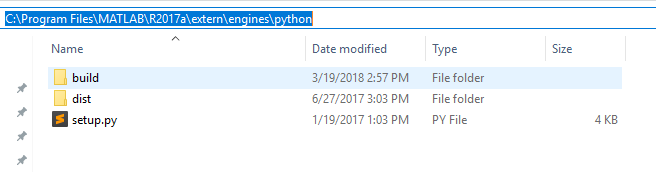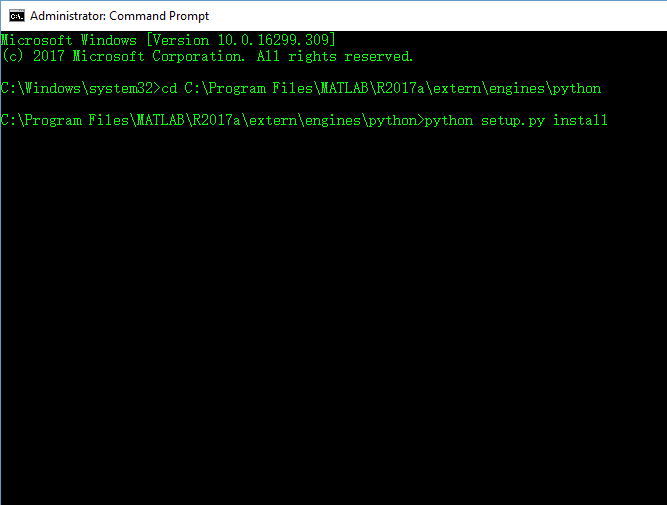• python中使用a = a + a创建了一个新的变量a，覆盖了之前的变量a。而使用a += a 则是直接对原变量 a 进行操作。下面通过几个例子进行说明： 例一 a = [1,2,3] a+=a print(a) 输出： [1,2,3,1,2,3] 例二 a = ...
python中使用a = a + a创建了一个新的变量a，覆盖了之前的变量a。而使用a += a 则是直接对原变量 a 进行操作。下面通过几个例子进行说明：
例一
a = [1,2,3]
a+=a
print(a)
输出：
[1,2,3,1,2,3]
例二
a = [1,2,3]
a = a + a
print(a)
输出：
[1,2,3,1,2,3]

从上面两个例子似乎并不能看出任何区别，下面再看两个例子
例三
a = [1,2,3]
arr += arr

print(a)
输出
[1,2,3,1,2,3]
例四
a = [1,2,3]
arr = arr + arr

print(a)
输出：
[1,2,3]

通过例三例四想必大家都能明白=与+=的区别了。=的使用会直接创建一个新的变量，而+=则是直接对原变量进行操作。
展开全文语法 = +=
• python计算功能很强大 计算a的b次方： a ** b
python计算功能很强大
计算a的b次方：
a ** b

展开全文计算
• ## 如何在Python中调用MATLAB

万次阅读 多人点赞 2018-03-21 10:16:28
鉴于实验内容需要，不得不找点办法MATLAB和Python之间建个接口，从Python中调用MATLAB脚本或者是MATLAB的函数。内容不是很难，毕竟现成的接口已经有了，这儿记录一下API使用的一些事项。 注：本篇使用的是...
最近在实验室做点杂活，一点声音的生成、录音、处理工作。鉴于实验内容需要，不得不找点办法在MATLAB和Python之间建个接口，从Python中调用MATLAB脚本或者是MATLAB的函数。内容不是很难，毕竟现成的接口已经有了，在这儿记录一下API使用的一些事项。
注：本篇使用的是MATLAB R2017a，windows 10系统。
相关链接
https://www.mathworks.com/help/matlab/matlab-engine-for-python.html  https://www.mathworks.com/help/matlab/matlab_external/get-started-with-matlab-engine-for-python.html
API的安装
MATLAB在安装的过程中已经准备好了相关文档。根据教程，首先要找到root\R2017a\extern\engines\python文件夹。这里的root指的是MATLAB安装的根目录。对于笔者的电脑，我的全路径就是C:\Program Files\MATLAB\R2017a\extern\engines\python。打开这个文件夹，可以看见下面这几个文件。其中setup.py是我们想要用的文件。定位到它之后，我们在command window里面转到这个文件夹，然后执行安装。（启动command window的时候必须是管理员模式，否则可能会报“没有权限”的错误）
cd C:\Program Files\MATLAB\R2017a\extern\engines\python
python setup.py install不出差错的话，到这里安装过程就结束了。如果你使用的是Python的虚拟环境，那么记得在安装前先将虚拟环境启动。
基本调用
这个API调用非常简单。在Python中，导入如下两个module就能实现MATLAB的全部控制：
import matlab
import matlab.engine
其中，matlab包含的是一些数据形式，比如int8，int16之类。matlab.engine负责程序的启动。
engine = matlab.engine.start_matlab() # Start MATLAB process
engine = matlab.engine.start_matlab("-desktop") # Start MATLAB process with graphic UI
上面两行代码，第一行指示MATLAB在后端运行；第二行则会启动MATLAB的图形界面窗口。调用任何MATLAB函数都通过engine变量来实现。笔者自己调用了一些声音工具箱中的函数，一点问题都没有。
>>> engine.sqrt(2.)
1.4142135623730951
变量兼容性
笔者自己尝试的时候遇到很多变量类型不正确这样的提示，因为MATLAB函数对于输入数据的类型有着比较严格的要求，比如log2对于int8类型输入就会报错。double类型是MATLAB里面用得最多的数据型，所以一般可以将python的数据转成MATLAB兼容的double类型来解决问题。举个例子，Python的list类型变量只需加上matlab.double就可以完成转换。
>>> engine.sqrt([1.,2.,3.,4.,5.])
# Some error
>>> engine.sqrt(matlab.double([1.,2.,3.,4.,5.]))
matlab.double([[1.0,1.4142135623730951,1.7320508075688772,2.0,2.23606797749979]])
调用MATLAB脚本及自定义函数
调用脚本和自定义函数的过程也几乎一样，也是从变量engine中去调用。值得注意的是，需要保证你的函数或脚本就在当前的工作路径下，否则engine会找不到文件而报错。
比方说，笔者当前的工作路径下有两个文件:gaussian_pulse.m和py_matlab.py。
gaussian_pulse.m中的内容是这样的：
function out = gaussian_pulse(fs, fc, repeat)
N = 882;
t = 0:1/fs:(N-1)/fs;
yi = gauspuls(t,fc,1/8);
temp = yi;
for i = 1:repeat-1
temp = cat(2, temp,yi);
end
out = temp;
end
在py_matlab.py中，我定义了engine并调用这个函数：
engine = matlab.engine.start_matlab()
signal = engine.gaussian_pulse(matlab.double(), matlab.double(), matlab.double())
注意到每一个输入参数我都强制性进行了转换确保万无一失。加上方括号也是因为MATLAB的格式需求。
指定输出个数
默认情况下，API认为接收函数返回结果的参数有1个。这会导致没有返回值的函数在被调用时报错：“Too many output arguments”或是其他类似的信息。我们可以人为指定输出参数为0个来避免这样的错误。
>>> playblocking(player) # playblocking is a MATLAB function with no returns
Error using playblocking
Too many output arguments
>>> playblocking(player, nargout=0)
# Success!

True 与 False
在MATLAB里面逻辑值是true与false，但是在Python里面它们是大写的True和False。毕竟我们还是在Python环境下编程，所以如果调用某个函数需要用到逻辑值，遵循Python的书写格式。
后记
官方文档里还有一些其他非常好的教程，包括标准输出/错误信息重导向、句柄的使用、画图等等。鉴于笔者工作的原因这些没有涉及到，有需要的朋友可以从笔者在开篇提供的网站中找到答案。
展开全文MATLAB
• numpy矩阵我们十分常用，但有时候我们会将矩阵转化为数组，方法很简单，直接矩阵名后加 .A 即可。 2. 演示 #!/usr/bin/env python3 # -*- coding: utf-8 -*- # @Time : 2019/2/21 19:13 # @Author : Arrow and...
1. 概述
在numpy中矩阵我们十分常用，但有时候我们会将矩阵转化为数组，方法很简单，直接在矩阵名后加 .A 即可。
2. 演示
#!/usr/bin/env python3
# -*- coding: utf-8 -*-
# @Time    : 2019/2/21 19:13
# @Author  : Arrow and Bullet
# @FileName: .A.py
# @Software: PyCharm
# @Blog    ：https://blog.csdn.net/qq_41800366
from numpy import *

matTest = mat([1, 2])
print(matTest, type(matTest))  # 结果：[[1 2]] <class 'numpy.matrixlib.defmatrix.matrix'>

matTestToArr = matTest.A
print(matTestToArr, type(matTestToArr))  # 结果：[[1 2]] <class 'numpy.ndarray'>

打印结果如下：
# [[1 2]] <class 'numpy.matrixlib.defmatrix.matrix'>
# [[1 2]] <class 'numpy.ndarray'>

希望能够帮助到大家，有什么问题可以 直接评论即可，喜欢有用的话可以点个赞让更多的人看到，如果不够详细的话也可以说，我会及时回复的。
展开全文• 欢迎一起学习研究 python(迎娶白富美!!!): 556993881+= 是 + 的一种升级版本, 具有能把执行后的结果再写回传递来的变量本身的功能, 可变变量自身有比不可变变量多一个魔法方法__iadd__举例:+=In : def selfAdd(a)...
• python列表随机选择Python random module provides an inbuilt method choice() has an ability to select a random item from the list and other sequences. Using the choice() method, either a single random...列表 人工智能 java 机器学习
• ## python中return的用法

万次阅读 多人点赞 2019-07-02 16:45:19
python中return的用法 1、return语句就是把执行结果返回到调用的地方，并把程序的控制权一起返回 程序运行到所遇到的第一个return即返回（退出def块），不会再运行第二个return。 例如： def haha(x,y): if x==y: ...return
• ## 如何在Python中使用断点调试

万次阅读 多人点赞 2018-10-18 22:11:59
实际上没人能一次就写出完美的代码，除了我。但是世界上只有一个我。 -- 林纳斯·托瓦兹（Linux 之父） ...而实际开发，调试代码通常要花费比新编写代码更多的时间。所谓“行百里者半于九十”，这句...
• 与||，在python中是没有这两个运算符的，取而代之的是英文的and和or。其他的运算符则没有发生变动。 a.any()函数的官方解释是： Test whether any elements of an array evaluate to True along an axis。 也就是说...
• ## python中全局变量与局部变量

万次阅读 多人点赞 2019-03-01 15:27:17
python中全局变量与局部变量 标签： python 局部变量就是定义一个函数体内部的变量 全局变量是定义外面的变量 比如： a = 1 def f(): b = 2 其中a就是全局变量，而b是局部变量。局部变量只函数体内部...
• ## 如何在python中调用C语言代码

万次阅读 多人点赞 2017-10-16 22:58:38
开发者有三种方法可以自己的Python代码来调用C编写的函数-ctypes，SWIG，Python/C API。每种方式也都有各自的利弊。 首先，我们要明确为什么要在Python中调用C？ 常见原因如下： - 你要提升代码的运行速度，...c语言 扩展
• python中三角函数 Python三角函数/方法 (Python Trigonometric functions/methods) In python programming language, there are some of the built-in functions which are defined in math module – they can be ...linux java 数据分析 os
• ## Python中产生随机数

万次阅读 多人点赞 2019-07-09 18:42:27
Python产生随机数： 一.Python自带的random库 1.参生n--m范围内的一个随机数: random.randint(n,m) 2.产生0到1之间的浮点数: random.random() 3.产生n---m之间的浮点数: random.uniform(1.1,5.4) 4.产生从n...
• ## python中取余%

万次阅读 2019-07-17 13:20:09
在Python中，取余的计算公式与别的语言并没有什么区别：r=a-n*[a//n]，其中a是被除数，n是除数。 这其中会涉及到被除数或者除数是负数。但只要知道其中的原理，就都可以解决。 其中如果除数是负数，那么取得的结果...程序员 编程
• ## python中的[:-1]和[::-1]

万次阅读 多人点赞 2018-08-12 11:28:56
a='python' b=a[::-1] print(b) #nohtyp c=a[::-2] print(c) #nhy #从后往前数的话，最后一个位置为-1 d=a[:-1] #从位置0到位置-1之前的数 print(d) #pytho e=a[:-2] #从位置0到位置-2之前的数 print(e) #pyth 2、...
• Python 的range(),以及numpy包中的arange()函数 range()函数 函数说明： range(start, stop[, step]) -&amp;amp;amp;gt; range object，根据start与stop指定的范围以及step设定的步长，生成一个序列。 参数...range numpy 函数
• Python 爬虫获取某个网页所有的a标签的超链接网址
• python中使用递归实现斐波那契数列 python中使用递归实现斐波那契数列 先来了解一下 斐波那契数列（Fibonacci sequence），又称黄金分割数列、因数学家莱昂纳多·斐波那契（Leonardoda Fibonacci）以兔子繁殖为例子...算法
• python列表查找值Hi Folks! In this article, we will have a look at the various ways to find the average... 本文，我们将介绍在Python List查找列表平均值的各种方法 。 In general, an average is a va...
• ## python中arange()和linspace()区别

万次阅读 多人点赞 2014-11-30 17:06:12
arange()类似于内置函数range()，通过指定开始值、终值和步长创建表示等差数列的一维数组，注意得到的结果数组不包含终值。 linspace()通过指定开始值、终值和元素个数创建表示等差数列的一维数组，可以通过...linspace numpy
• ## Python中输出百分数

千次阅读 2019-03-21 23:02:49
在python中输出百分数：例如99.99% a=99.99 print("%.2f%%" % a)#显示小数点后两位，其中%%代表输出%
• 在python中导入csv文件的数据为矩阵的方法 因为初学python和深度学习，因此总是导入一些csv，txt文件时遇到一些莫名其妙的错误或者问题，因此这里总结了一下我导入数据的办法，也供大家参考。 方法一 csv...csv 矩阵 数据
• 原文地址：... OpenCV有两个Python接口，老版本的cv模块使用OpenCV内置的数据类型，新版本的cv2模块使用NumPy数组。对于新版本的模块，可以通过下面方式导入：import cv2...opencv
• 假如我A文件夹中写了两个python文件a.py和b.py，文件b中有函数c，我a文件中这样写： from A.b import c ...我文件夹A中明明有b文件啊，为什么计算机找不到呢？怎么解决呢？（linux系统，终端运行）
• ## python中split函数

万次阅读 多人点赞 2019-09-08 21:44:32
Python中split()：split() 方法可以实现将一个字符串按照指定的分隔符切分成多个子串，这些子串会被保存到列表（不包含分隔符），作为方法的返回值反馈回来。 s.split(sep,maxsplit) str：表示要进行分割的字符...
• Python is a very expressive language that provides different structures to easy developers work. The list is one of the most popular data structures provided by the python. In a regular workflow, we a...
• ## XGBoost：在Python中使用XGBoost

万次阅读 多人点赞 2015-07-06 11:27:44
在Python中使用XGBoost下面将介绍XGBoost的Python模块，内容如下： * 编译及导入Python模块 * 数据接口 * 参数设置 * 训练模型l * 提前终止程序 * 预测A walk through python example for UCI Mushroom ...xgboost
• 发表于 http://liamchzh.0fees.net/?p=234&amp;i=1 一次偶然的机会，发现python中list非常有意思。 先看一段代码 [py]array = [0二维数组 定义 数组
• 在python的程序设计，输出含有双引号的字符串： a="Albert Einstein once said," b="A person who never made a mistake never tried anything new." full=a+"\""+b+"\"" print(full) full=a+'"'+b+'"' print...
• Protobuf在Python中的使用 本文原创自esbat的博客,转载请注明出处 protocol buffer的安装 $wget http://protobuf.googlecode.com/files/protobuf-2.4.0a.tar.gz$ tar zxvf protobuf-2.4.0a.tar.gz \$ cd protobuf......

# 在python中apython 订阅